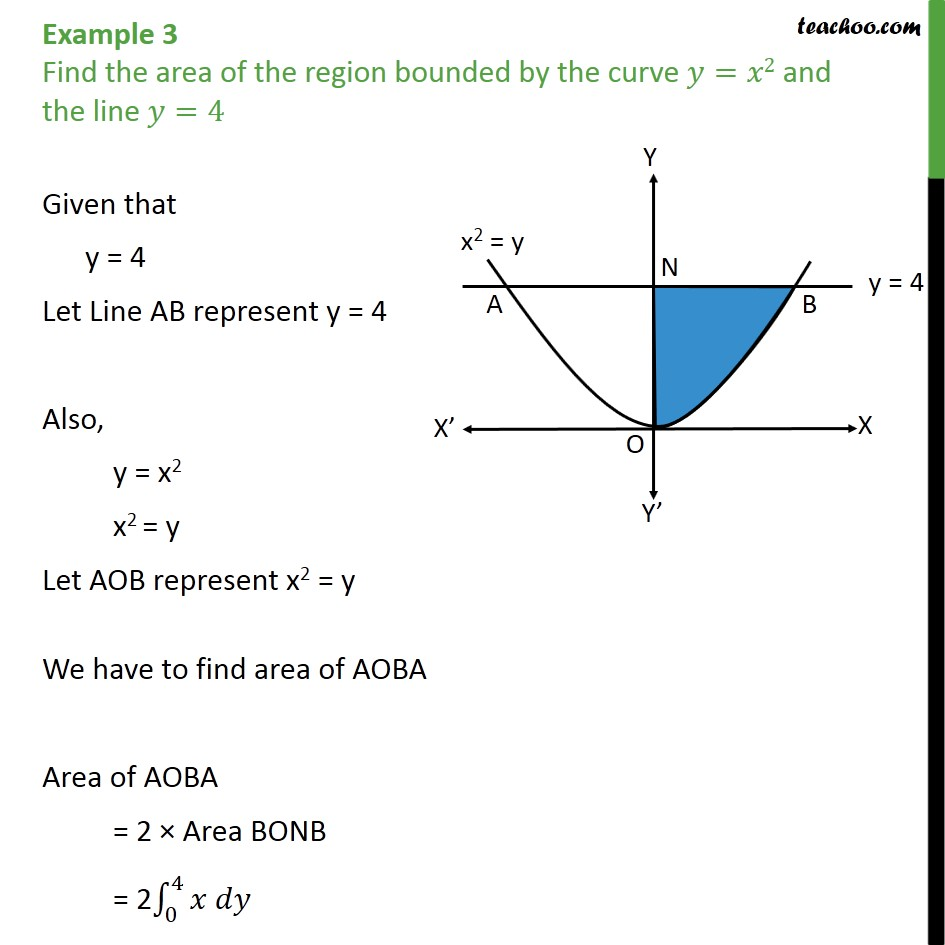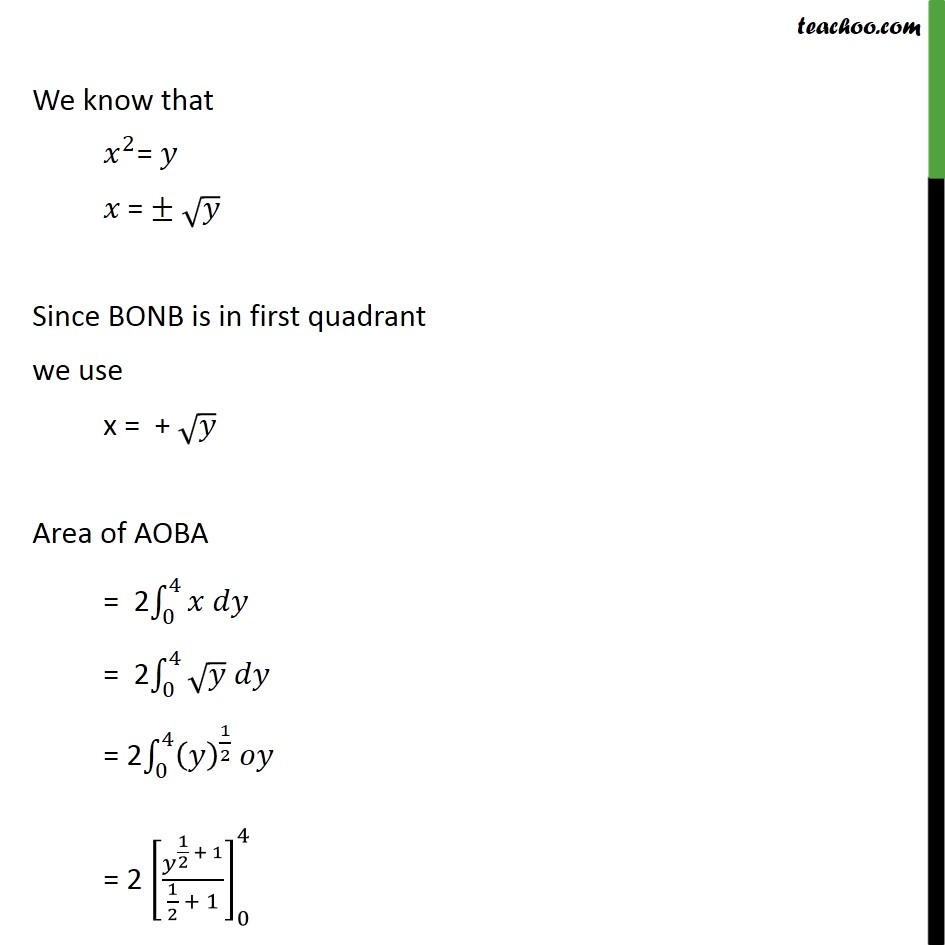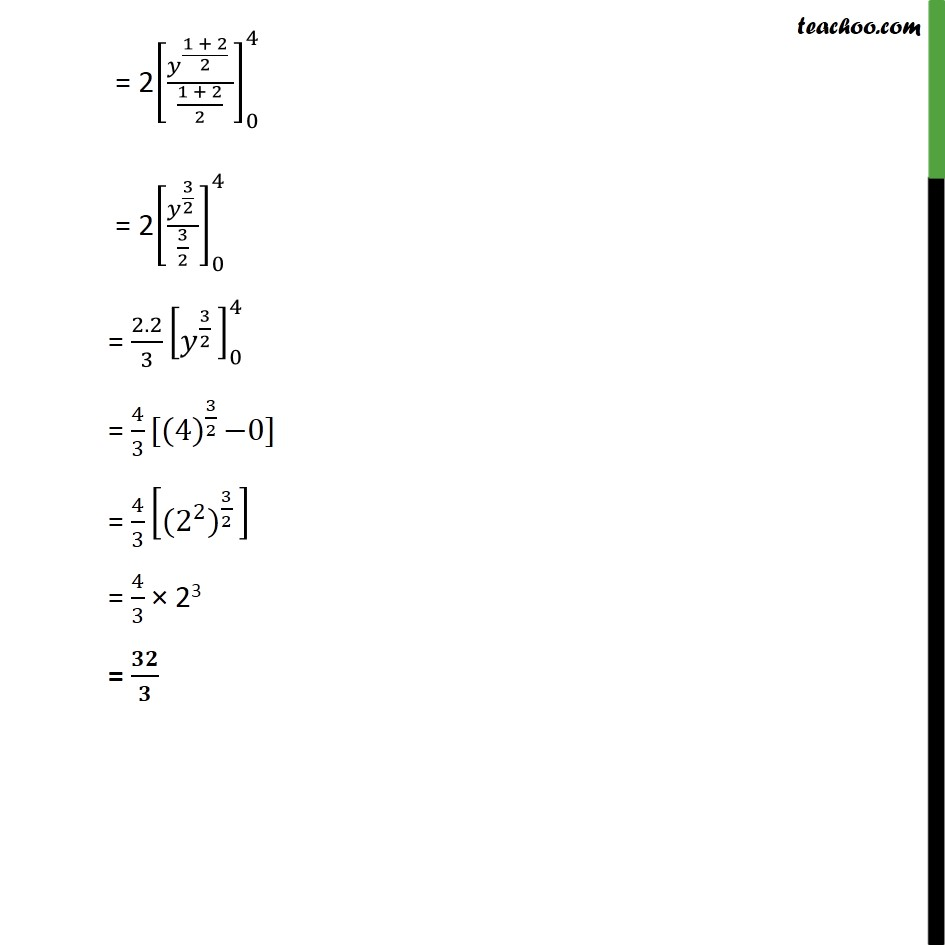Area bounded by curve and horizontal or vertical line

Chapter 8 Class 12 Application of Integrals
Concept wiseLearn in your speed, with individual attention - Teachoo Maths 1-on-1 Class

### Transcript

Question 1 Find the area of the region bounded by the curve = 2 and the line =4 Given that y = 4 Let Line AB represent y = 4 Also, y = x2 x2 = y Let AOB represent x2 = y We have to find area of AOBA Area of AOBA = 2 Area BONB = 2 0 4 We know that 2 = = Since BONB is in first quadrant we use x = + Area of AOBA = 2 0 4 = 2 0 4 = 2 0 4 1 2 = 2 1 2 + 1 1 2 + 1 0 4 = 2 1 + 2 2 1 + 2 2 0 4 = 2 3 2 3 2 0 4 = 2.2 3 3 2 0 4 = 4 3 [(4) 3 2 0] = 4 3 ( 2 2 ) 3 2 = 4 3 23 =